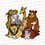# Elementary Techniques used in the IMO (International Mathematical Olympiad) - Invariants

Invariants Part $2$

Actually, in my honest opinion, this should be post $0$. Moving on..

In this post, we will introduce another method, commonly seen in invariant problems.

Let us begin with our first example:

Example 0 : $23$ friends wants to play hockey. However, to ensure that the game is fair play, they choose a referee and the rest of the team splits into $2$ teams of $11$ people each. However, because they are a bit eccentric, they want to do this splitting such that the total weight of each team is the same. Suppose now for simplicity sake that they all have integer weights, and we know that this division is always possible regardless of who is the referee. Show that they all have the same weight.

Solution: Wow, that is a really really long question and to help us understand the context and meaning, we rewrite this in mathematical equations. Let $w_1, w_2, \ldots, w_23$ be the weights of the $23$ people. Also, let $S = w_1 + w_2 + \ldots + w_23$, the motivation behind this definition is that choosing a referee is the same as removing a person with weight $w_i$ and split the rest into $2$ teams of weight $k$ each. Now, we can write everything in this compact form: $S - w_i = 2k (*)$. Remember what we said about parity? Clearly since the RHS is even, $w_i$ and $S$ needs to have the same parity. And since $w_i$ was completely arbitrary, they are all odd or even.

Being odd or even only gives us one clue on how to proceed, which is whether $2$ divides it or not. Because we want to work with simplified things, consider what happens if we divide those that are even by $2$. For instance, if all $w_i$ are even, if we let $q_i = \frac{w_i}{2}$, does this simplify the problem to some extent? Well, clearly by definition all $q_i$ are integers, and writing a equation, $\frac{S}{2} - \frac{q_i} = 2\frac{k}{2}$ which is unmistakably $(*)$ so $q_i$ is a new list of weights that also satisfy the conditions. Analogously, we can conclude for $w_i$ being odd (don't forget this case!), we can let $q_i = w_i - 1$.

Here's the important bit. If the $w_i$ at the beginning were not all $0$, then the sum of the weights $q_i$ is strictly smaller. We can always repeat this step (this is sort of an algorithm-like approach - another method that works like induction; you use it if you do not have any idea how to proceed) and reduce the sum of the weights so eventually we will reach a list of only zeroes. Now we backtrack to see what this implies. So since we are able to do this, we conclude the numbers in the original list were all equal, as desired. □

Please do NOT read this until you attempted the problem in the previous post.

Solution to that problem: Because we have square roots and $a+b$ and $a-b$ on the numerator, which reminds me of difference of squares, let us square the expression to get rid of the nasty square roots. So remark that:

$a^2 + b^2 = (\frac{a+b}{\sqrt{2}})^2 + (\frac{a-b}{\sqrt{2}})^2$

Now, the sum of squares of the numbers in the triple is invariant under the operation. Just notice now that the sum of squares of the first triple is $\frac{9}{2}$ and that of te second is $6 + 2 \sqrt{2}$ so the first triple can never be transformed into the second. ■Note by Anqi Li
7 years, 5 months ago

This discussion board is a place to discuss our Daily Challenges and the math and science related to those challenges. Explanations are more than just a solution — they should explain the steps and thinking strategies that you used to obtain the solution. Comments should further the discussion of math and science.

When posting on Brilliant:

• Use the emojis to react to an explanation, whether you're congratulating a job well done , or just really confused .
• Ask specific questions about the challenge or the steps in somebody's explanation. Well-posed questions can add a lot to the discussion, but posting "I don't understand!" doesn't help anyone.
• Try to contribute something new to the discussion, whether it is an extension, generalization or other idea related to the challenge.

MarkdownAppears as
*italics* or _italics_ italics
**bold** or __bold__ bold
- bulleted- list
• bulleted
• list
1. numbered2. list
1. numbered
2. list
Note: you must add a full line of space before and after lists for them to show up correctly
paragraph 1paragraph 2

paragraph 1

paragraph 2

[example link](https://brilliant.org)example link
> This is a quote
This is a quote
    # I indented these lines
# 4 spaces, and now they show
# up as a code block.

print "hello world"
# I indented these lines
# 4 spaces, and now they show
# up as a code block.

print "hello world"
MathAppears as
Remember to wrap math in $$ ... $$ or $ ... $ to ensure proper formatting.
2 \times 3 $2 \times 3$
2^{34} $2^{34}$
a_{i-1} $a_{i-1}$
\frac{2}{3} $\frac{2}{3}$
\sqrt{2} $\sqrt{2}$
\sum_{i=1}^3 $\sum_{i=1}^3$
\sin \theta $\sin \theta$
\boxed{123} $\boxed{123}$

Sort by:

Argh there are some typos. I wonder if Calvin can be nice enough to help me fix them? Thanks in advance :)

- 7 years, 5 months ago

Thanks

- 7 years, 5 months ago